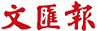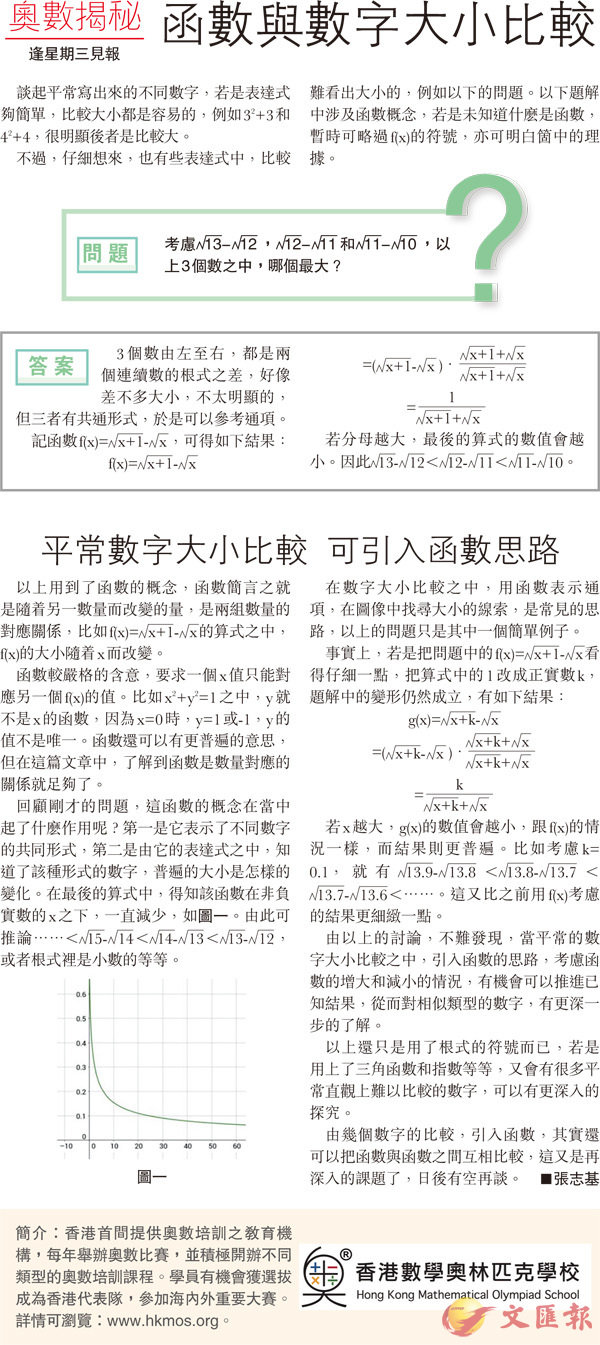首頁 > 文匯報 > 教育 > 正文

# 【奧數揭秘】函數與數字大小比較

2017-06-073個數由左至右，都是兩個連續數的根式之差，好像差不多大小，不太明顯的，但三者有共通形式，於是可以參考通項。

f(x)=　[x+1- x]

=　[x+1- x]　[( )．]　[x+1+ x]　　[x+1+ x]

=　[x+1+ x]　　

g(x)=　[x+k- x]

=　[x+k- x]　[( )．]　[x+k+ x]　　　[x+k+ x]

=　[x+k+ x]　　[k]